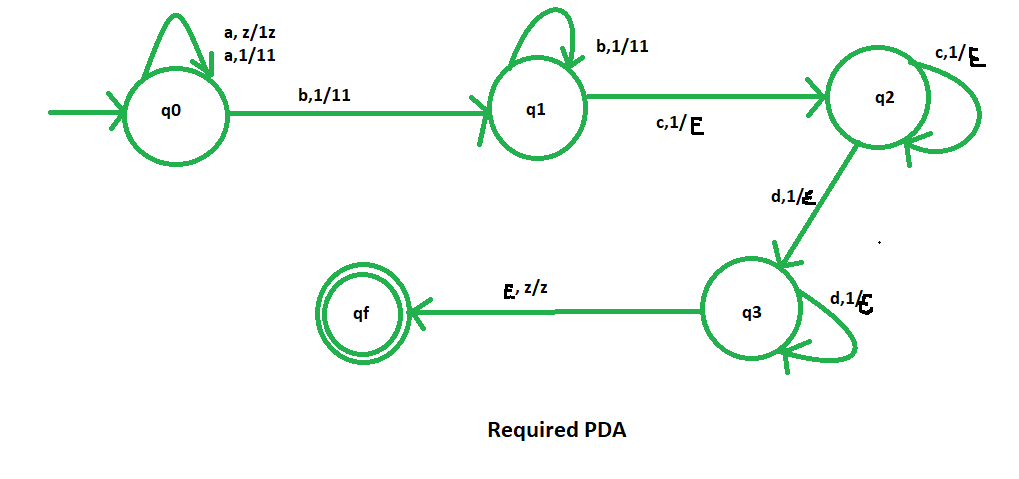Open in App
Not now

# NPDA for accepting the language L = {am bn cp dq | m+n=p+q ; m,n,p,q>=1}

• Last Updated : 28 Jun, 2018

Prerequisite – Pushdown automata, Pushdown automata acceptance by final state
Problem – Design a non deterministic PDA for accepting the language L = {| m + n = p + q : m, n, p, q>=1}, i.e.,

L = {abcd, abbcdd, abbccd, abbbccdd, ......}

In each of the string, the total number of ‘a’ and ‘b’ are equal to the total number of ‘c’ and ‘d’.

Explanation –
Here, we need to maintain the order of ‘a’, ‘b’, ‘c’ and ‘d’. Thus, we need a stack along with the state diagram. The count of ‘a’, ‘b’, ‘c’ and ‘d’ is maintained by the stack. We will take 2 stack alphabets:= { 1, z }

Where,= set of all the stack alphabet
z = stack start symbol

Approach used in the construction of PDA –
As we want to design a NPDA, thus every time ‘a’, ‘b’, ‘c’ and ‘d’ will comes in proper order. When ‘a’ and ‘b’ comes then we will push ‘1’ into the stack. After that, when ‘c’ and ‘d’ comes then pop ‘1’ from the stack each time. So, at the end if the stack becomes empty then we can say that the string is accepted by the PDA.

Stack transition functions –(q0, a, z)(q0, 1z)(q0, a, 1)(q0, 11)(q0, b, 1)(q1, 11)(q1, b, 1)(q1, 11)(q1, c, 1)(q2,)(q2, c, 1)(q2,)(q2, d, 1)(q3,)(q3, d, 1)(q3,)(q3,, z)(qf, z)


Where, q0 = Initial state
qf = Final state= indicates pop operationSo, this is our required non deterministic PDA for accepting the language L = {| m + n = p + q ; m, n, p, q>=1 }.

My Personal Notes arrow_drop_up